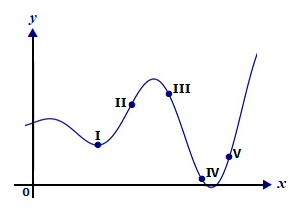# The following figure shows the graph of y = f(x). At one of the labeled points, dy/dx and...

## Question:

The following figure shows the graph of {eq}y = f(x) {/eq}. At one of the labeled points, {eq}\displaystyle\frac{dy}{dx} {/eq} and {eq}\displaystyle\frac{d^{2}y}{dx^{2}} {/eq} are both positive. Which point is it?## Function:

The function is increasing at the point at which the first derivative is positive, decreasing at the point at which the first derivative is negative, and concavity is upward at the point at which the second derivative is positive, the concavity is downward at the point at which the second derivative is negative.

We know that the function is increasing at the point at which the first derivative is positive and concavity is upward at the point at which the second derivative is positive. From the given graph we observe that at point {eq}V {/eq}, the graph is increasing as well as concave upwards, hence the required point is {eq}V. {/eq}